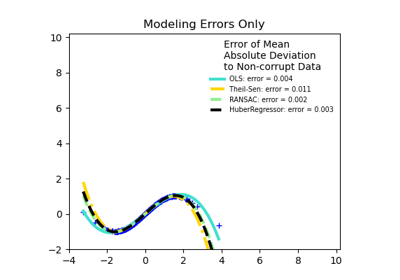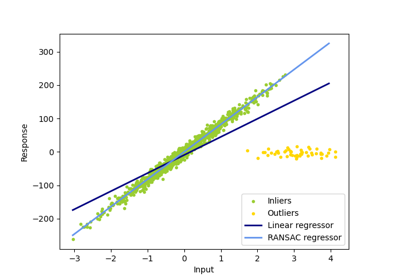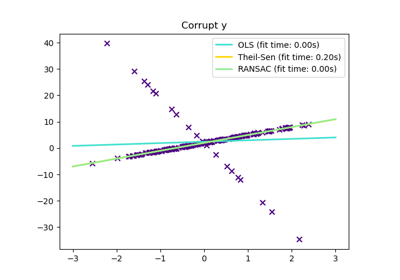# sklearn.linear_model.RANSACRegressor¶

class sklearn.linear_model.RANSACRegressor(estimator=None, *, min_samples=None, residual_threshold=None, is_data_valid=None, is_model_valid=None, max_trials=100, max_skips=inf, stop_n_inliers=inf, stop_score=inf, stop_probability=0.99, loss='absolute_error', random_state=None, base_estimator='deprecated')[source]

RANSAC (RANdom SAmple Consensus) algorithm.

RANSAC is an iterative algorithm for the robust estimation of parameters from a subset of inliers from the complete data set.

Read more in the User Guide.

Parameters:
estimatorobject, default=None

Base estimator object which implements the following methods:

• fit(X, y): Fit model to given training data and target values.

• score(X, y): Returns the mean accuracy on the given test data, which is used for the stop criterion defined by stop_score. Additionally, the score is used to decide which of two equally large consensus sets is chosen as the better one.

• predict(X): Returns predicted values using the linear model, which is used to compute residual error using loss function.

If estimator is None, then LinearRegression is used for target values of dtype float.

Note that the current implementation only supports regression estimators.

min_samplesint (>= 1) or float ([0, 1]), default=None

Minimum number of samples chosen randomly from original data. Treated as an absolute number of samples for min_samples >= 1, treated as a relative number ceil(min_samples * X.shape) for min_samples < 1. This is typically chosen as the minimal number of samples necessary to estimate the given estimator. By default a sklearn.linear_model.LinearRegression() estimator is assumed and min_samples is chosen as X.shape + 1. This parameter is highly dependent upon the model, so if a estimator other than linear_model.LinearRegression is used, the user must provide a value.

residual_thresholdfloat, default=None

Maximum residual for a data sample to be classified as an inlier. By default the threshold is chosen as the MAD (median absolute deviation) of the target values y. Points whose residuals are strictly equal to the threshold are considered as inliers.

is_data_validcallable, default=None

This function is called with the randomly selected data before the model is fitted to it: is_data_valid(X, y). If its return value is False the current randomly chosen sub-sample is skipped.

is_model_validcallable, default=None

This function is called with the estimated model and the randomly selected data: is_model_valid(model, X, y). If its return value is False the current randomly chosen sub-sample is skipped. Rejecting samples with this function is computationally costlier than with is_data_valid. is_model_valid should therefore only be used if the estimated model is needed for making the rejection decision.

max_trialsint, default=100

Maximum number of iterations for random sample selection.

max_skipsint, default=np.inf

Maximum number of iterations that can be skipped due to finding zero inliers or invalid data defined by is_data_valid or invalid models defined by is_model_valid.

New in version 0.19.

stop_n_inliersint, default=np.inf

Stop iteration if at least this number of inliers are found.

stop_scorefloat, default=np.inf

Stop iteration if score is greater equal than this threshold.

stop_probabilityfloat in range [0, 1], default=0.99

RANSAC iteration stops if at least one outlier-free set of the training data is sampled in RANSAC. This requires to generate at least N samples (iterations):

N >= log(1 - probability) / log(1 - e**m)


where the probability (confidence) is typically set to high value such as 0.99 (the default) and e is the current fraction of inliers w.r.t. the total number of samples.

lossstr, callable, default=’absolute_error’

String inputs, ‘absolute_error’ and ‘squared_error’ are supported which find the absolute error and squared error per sample respectively.

If loss is a callable, then it should be a function that takes two arrays as inputs, the true and predicted value and returns a 1-D array with the i-th value of the array corresponding to the loss on X[i].

If the loss on a sample is greater than the residual_threshold, then this sample is classified as an outlier.

New in version 0.18.

random_stateint, RandomState instance, default=None

The generator used to initialize the centers. Pass an int for reproducible output across multiple function calls. See Glossary.

base_estimatorobject, default=”deprecated”

Use estimator instead.

Deprecated since version 1.1: base_estimator is deprecated and will be removed in 1.3. Use estimator instead.

Attributes:
estimator_object

Best fitted model (copy of the estimator object).

n_trials_int

Number of random selection trials until one of the stop criteria is met. It is always <= max_trials.

Boolean mask of inliers classified as True.

n_skips_no_inliers_int

Number of iterations skipped due to finding zero inliers.

New in version 0.19.

n_skips_invalid_data_int

Number of iterations skipped due to invalid data defined by is_data_valid.

New in version 0.19.

n_skips_invalid_model_int

Number of iterations skipped due to an invalid model defined by is_model_valid.

New in version 0.19.

n_features_in_int

Number of features seen during fit.

New in version 0.24.

feature_names_in_ndarray of shape (n_features_in_,)

Names of features seen during fit. Defined only when X has feature names that are all strings.

New in version 1.0.

HuberRegressor

Linear regression model that is robust to outliers.

TheilSenRegressor

Theil-Sen Estimator robust multivariate regression model.

SGDRegressor

Fitted by minimizing a regularized empirical loss with SGD.

References

Examples

>>> from sklearn.linear_model import RANSACRegressor
>>> from sklearn.datasets import make_regression
>>> X, y = make_regression(
...     n_samples=200, n_features=2, noise=4.0, random_state=0)
>>> reg = RANSACRegressor(random_state=0).fit(X, y)
>>> reg.score(X, y)
0.9885...
>>> reg.predict(X[:1,])
array([-31.9417...])


Methods

 fit(X, y[, sample_weight]) Fit estimator using RANSAC algorithm. get_params([deep]) Get parameters for this estimator. Predict using the estimated model. score(X, y) Return the score of the prediction. set_params(**params) Set the parameters of this estimator.
fit(X, y, sample_weight=None)[source]

Fit estimator using RANSAC algorithm.

Parameters:
X{array-like, sparse matrix} of shape (n_samples, n_features)

Training data.

yarray-like of shape (n_samples,) or (n_samples, n_targets)

Target values.

sample_weightarray-like of shape (n_samples,), default=None

Individual weights for each sample raises error if sample_weight is passed and estimator fit method does not support it.

New in version 0.18.

Returns:
selfobject

Fitted RANSACRegressor estimator.

Raises:
ValueError

If no valid consensus set could be found. This occurs if is_data_valid and is_model_valid return False for all max_trials randomly chosen sub-samples.

get_params(deep=True)[source]

Get parameters for this estimator.

Parameters:
deepbool, default=True

If True, will return the parameters for this estimator and contained subobjects that are estimators.

Returns:
paramsdict

Parameter names mapped to their values.

predict(X)[source]

Predict using the estimated model.

This is a wrapper for estimator_.predict(X).

Parameters:
X{array-like or sparse matrix} of shape (n_samples, n_features)

Input data.

Returns:
yarray, shape = [n_samples] or [n_samples, n_targets]

Returns predicted values.

score(X, y)[source]

Return the score of the prediction.

This is a wrapper for estimator_.score(X, y).

Parameters:
X(array-like or sparse matrix} of shape (n_samples, n_features)

Training data.

yarray-like of shape (n_samples,) or (n_samples, n_targets)

Target values.

Returns:
zfloat

Score of the prediction.

set_params(**params)[source]

Set the parameters of this estimator.

The method works on simple estimators as well as on nested objects (such as Pipeline). The latter have parameters of the form <component>__<parameter> so that it’s possible to update each component of a nested object.

Parameters:
**paramsdict

Estimator parameters.

Returns:
selfestimator instance

Estimator instance.

## Examples using sklearn.linear_model.RANSACRegressor¶Robust linear estimator fitting

Robust linear estimator fittingRobust linear model estimation using RANSAC

Robust linear model estimation using RANSACTheil-Sen Regression

Theil-Sen Regression